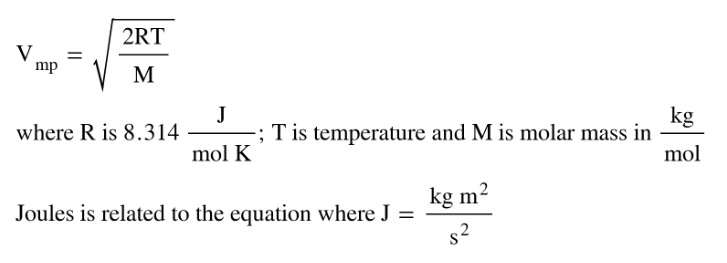# Problem: Calculate the most probable speed of CO molecules at 315 K .

###### FREE Expert Solution
• The most probable speed can be calculated using the molar mass of the gas, temperature and the gas constant R:• Remember to use appropriate units to get the Vmp in m/s
• Vmp for CO will appear as:
87% (260 ratings)###### Problem Details

Calculate the most probable speed of CO molecules at 315 K .